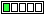All about flooble | fun stuff | Get a free chatterbox | Free JavaScript | Avatarsperplexus dot infoPennies = Nickels (Posted on 2009-10-08)A machine gives out five pennies for each nickel inserted into it. The machine also gives out five nickels for each penny.

Starting out with one penny, is it possible to use the machine in such a manner as to end up with an equal number of nickels and pennies?

As a bonus, is it possible to end up with exactly one dollar?

 No Solution Yet Submitted by K Sengupta Rating: 2.3333 (3 votes)Comments: ( Back to comment list | You must be logged in to post comments.)Equality| Comment 1 of 5

Let n be the number of nickels and p be the number of pennies, so that [n, p] represents the mixture of coins at some point.  Whenever you convert a nickel into 5 pennies, the result is [n, p] �� [n-1, p+5].  And likewise whenever you convert a penny into 5 nickels, the result is [n, p] �� [n+5, p-1].  In either case, the parity (oddness or evenness) of both values get reversed, since 1 and 5 are both odd.  Given the initial state of [0, 1] (one penny), where their parity is different, any set of transactions will always result in [n, p] have different parity.  Therefore n can never equal p.

(I'd like to get a hold of this machine, where can I find one?!)

 Posted by Jim Keneipp on 2009-10-08 13:08:29Please log in:

 Search: Search body:
Forums (0)